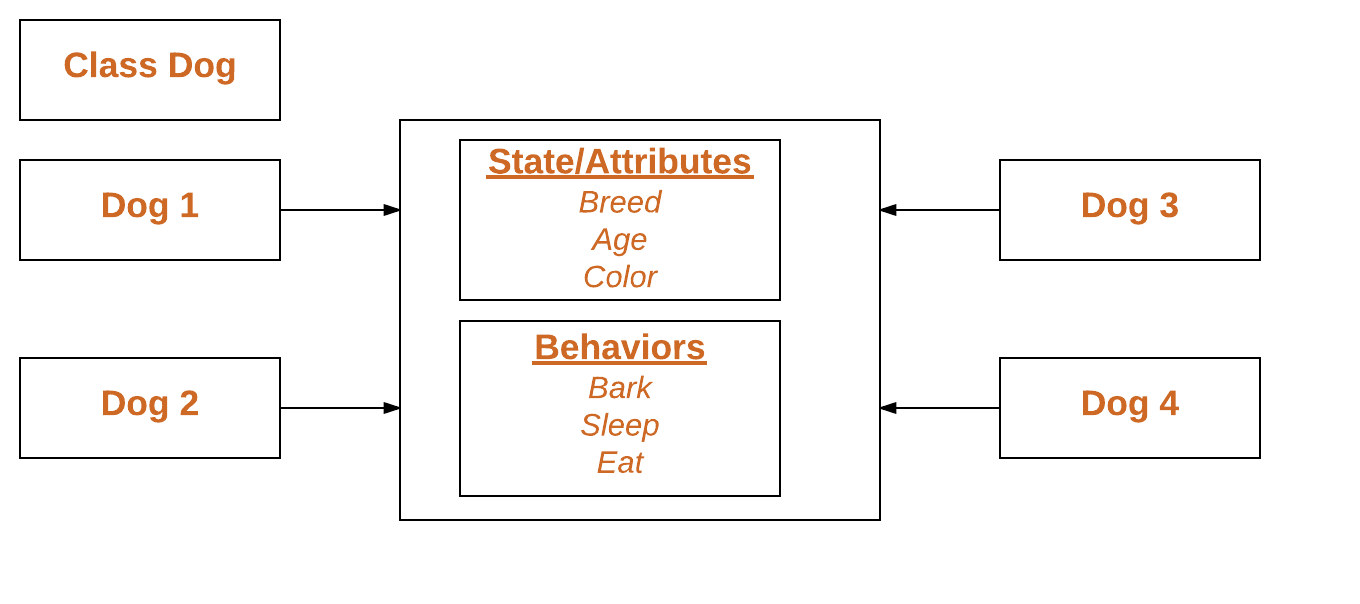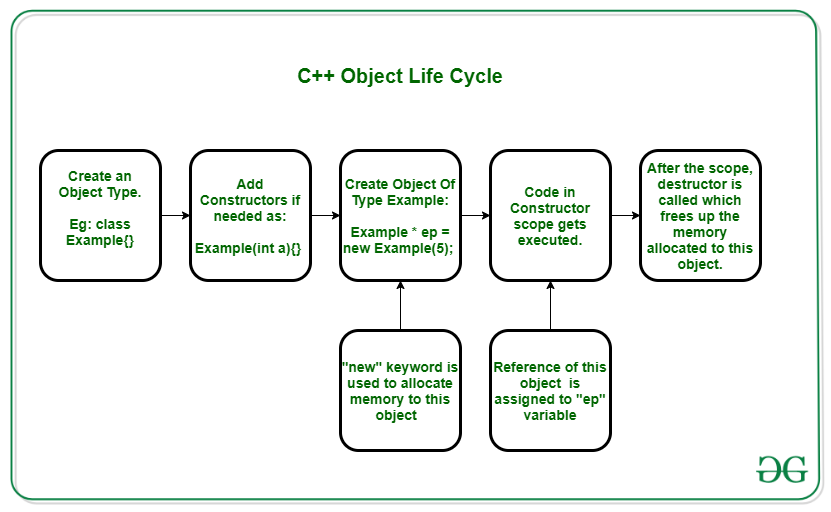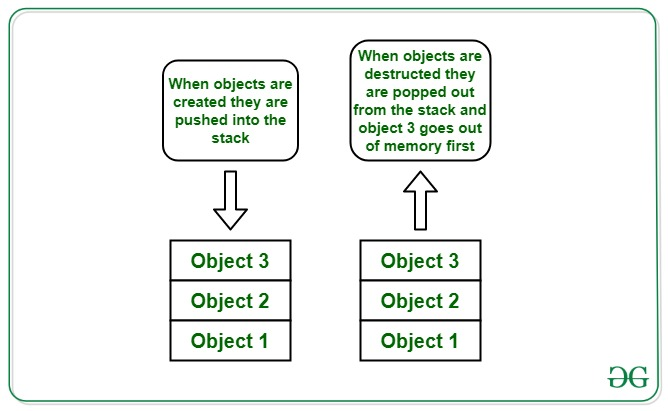# Life cycle of Objects in C++ with Example

• Last Updated : 04 Aug, 2021

In Object Oriented Programming, Objects are the instances of a class which has its own state(variables) and behavior(methods).Every class has two special methods related with creation and destruction of object of a class- constructors and destructors.

### C++ Object Life Cycle:There are various steps that need to be followed to complete the life cycle of an object:

1. First and foremost, some class is needed to define a class-based object. Therefore, an Example class is created in the above diagram.
2. A constructor constructs values of the class type. It is a member function whose name is the same as the class name. This process involves initializing data members and, frequently, allocating free store using new.
3. One can initialize the Example Object as created above. Initialization requires new keyword to be called, to allot some memory to this object.
4. One can use some logic in the constructor, which will be executed during initialization.
5. After execution is done, destructor is called. A destructor is a member function whose purpose is to destroy values of the class type. It is a member function whose name is preceded by the tilde(~) character.
6. During this whole life cycle, remember the following facts:
• A constructor is invoked when its associated type is used in a definition.
• Destructors are invoked implicitly when an object goes out of scope.
• Constructors and destructors do not have return types and cannot use return statements.

Below is the program for how constructor and destructors work in C++:

## C++

 `// C++ program to demonstrate the``// object allocation & deallocation` `#include ``using` `namespace` `std;` `class` `object {``public``:``    ``// Constructor``    ``object()``    ``{``        ``// Constructor has same name``        ``// as that of class name``        ``cout << ``"The object is created"``             ``<< ``"\n"``;``    ``}` `    ``// Destructor``    ``~object()``    ``{``        ``// Destructor has same name as``        ``// class and is preceded by ~ sign``        ``cout << ``"The object is destructed"``             ``<< ``"\n"``;``    ``}``};` `// Driver Code``int` `main()``{``    ``// Object creation``    ``object obj1;` `    ``return` `0;``}`

Output:

```The object is created
The object is destructed```

### When multiple objects are involved:When multiple objects are created from the same class, the construction of the objects takes place in the same way they are created. However, the destruction follows LIFO(Last In First Out) approach i.e., the object which was created first will be destructed last. Since, when working with a single class, every new object is independent of the previous one so the order of destruction doesn’t matter much. However, while working with inheritance, this LIFO order does make sense.

Below is the program to illustrate the same:

## C++

 `// C++ program to illustrate the``// constructor and destructor when``// multiple objects are created` `#include ``using` `namespace` `std;` `// obj Class``class` `obj {``public``:``    ``// Declare class variable to``    ``// keep count on objects``    ``static` `int` `obj_count;` `    ``// Constructor``    ``obj()``    ``{``        ``obj_count++;``        ``cout << ``"The obj - "``             ``<< obj_count``             ``<< ``" - is created"``             ``<< ``"\n"``;``    ``}` `    ``// Destructor``    ``~obj()``    ``{``        ``cout << ``"The obj - "``             ``<< obj_count``             ``<< ``" - is destructed"``             ``<< ``"\n"``;``        ``obj_count--;``    ``}``};` `// Static members are always defined``// outside of the class``int` `obj::obj_count = 0;` `// Driver Code``int` `main()``{``    ``// Creating objects``    ``obj obj1{};``    ``obj obj2{};` `    ``return` `0;``}`

Output:

```The obj - 1 - is created
The obj - 2 - is created
The obj - 2 - is destructed
The obj - 1 - is destructed```

### Behavior of object construction and destruction in case of inheritance:

Inheritance in C++ follows a IS-A approach. When a class B, inherits a class A, we say B IS-A. We say class B as derived class and class A as base class. Along with state and behaviors, class B also inherits constructor and destructor of the class A. There are some rules guiding inheritance of constructor and destructor.

• Derived class can not override or overload constructor and destructor of base class.
• The construction of base class takes place first, followed by the construction of derived class.
• The destruction of derived class takes place first.

Below is the program to illustrate the same:

## C++

 `// C++ program to demonstrates the``// object Allocation and deallocation``// during inheritance B stands for base``// class and D stands for derived class` `#include ``using` `namespace` `std;` `// Class B``class` `B {``public``:``    ``// Constructor``    ``B(``int` `b = 0)``        ``: _b(b)``    ``{``        ``cout << ``"constructor of base class "``             ``<< ``"created with value - "``             ``<< _b << ``'\n'``;``    ``}` `    ``// Destructor``    ``~B()``    ``{``        ``cout << ``"Destructor of base class "``             ``<< ``"called \n"``;``    ``}``    ``int` `_b;``};` `// Inherit class D from class B``class` `D : ``public` `B {` `public``:``    ``D(``int` `d)``        ``: _d(d)``    ``{``        ``// Default constructor of b``        ``// Is called automatically``        ``cout << ``"constructor of derived "``             ``<< ``" class created with value - "``             ``<< _d << ``'\n'``;``    ``}` `    ``// Overloaded Constructor``    ``D(``int` `b, ``int` `d)``        ``: B(b), _d(d)``    ``{``        ``cout << ``"constructor of derived class "``             ``<< ``"created with value - "``             ``<< _d << ``'\n'``;``    ``}` `    ``// Destructor``    ``~D()``    ``{``        ``cout << ``"Destructor of derived class "``             ``<< ``"called \n"``;``    ``}` `private``:``    ``int` `_d;``};` `// Driver Code``int` `main()``{``    ``// Object of class B``    ``B b(34);` `    ``// Objects of class D``    ``D d2(89);``    ``D d1(56, 78);` `    ``return` `0;``}`

Output:
constructor of base class created with value – 34
constructor of base class created with value – 0
constructor of derived class created with value – 89
constructor of base class created with value – 56
constructor of derived class created with value – 78
Destructor of derived class called
Destructor of base class called
Destructor of derived class called
Destructor of base class called
Destructor of base class called

### Why LIFO approach is used during object destruction?

Since the child class is inheriting states and behaviors from the parent class, so it makes sense that first all the work of child class should be finished and then only the object of the base class must be destroyed. Suppose, child class accesses a state from parent class but the parent object has been destructed, in this case, an error would occur and hence LIFO order is followed in destruction. This goes well even while working with multiple objects of single class.

My Personal Notes arrow_drop_up